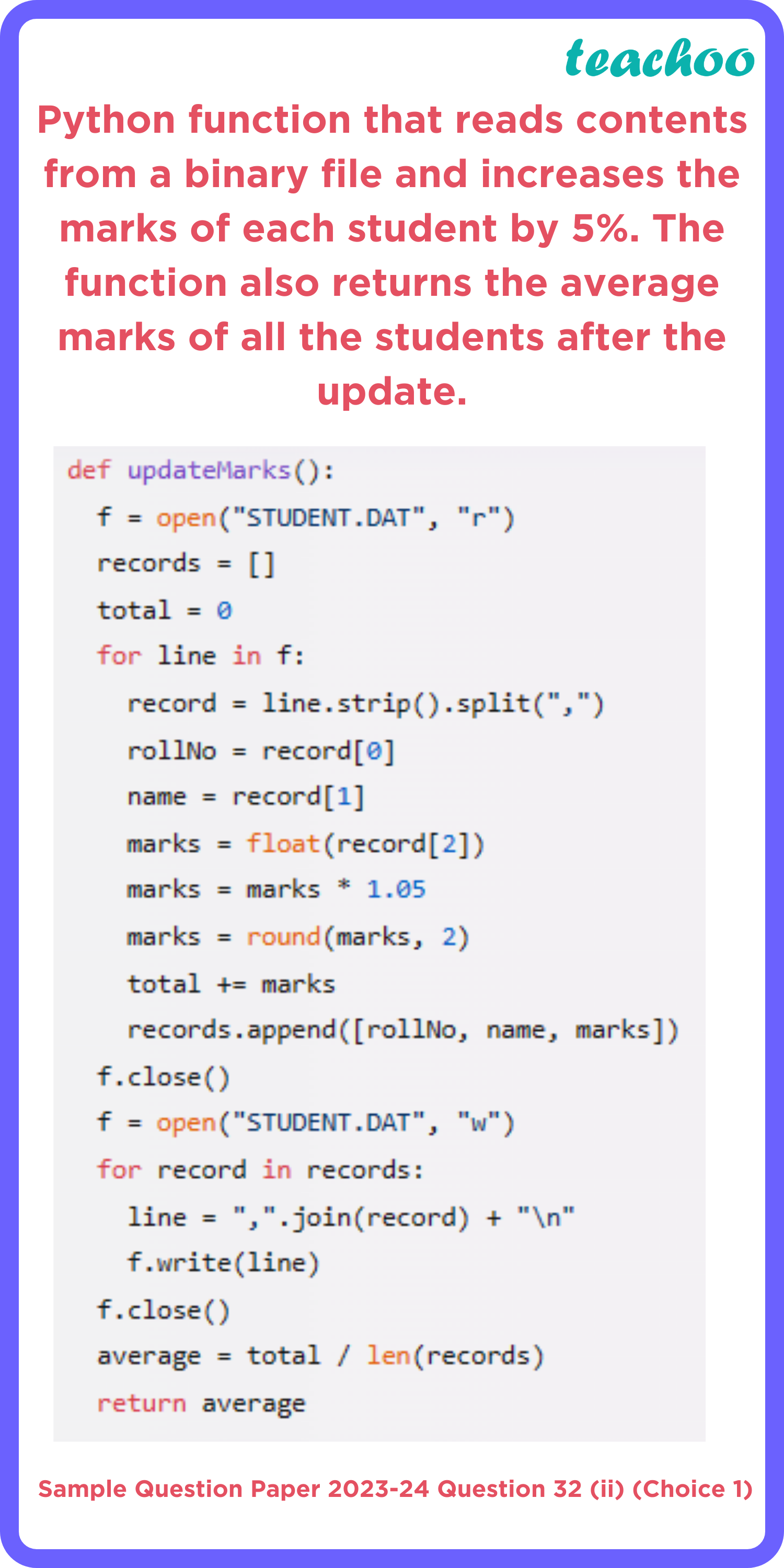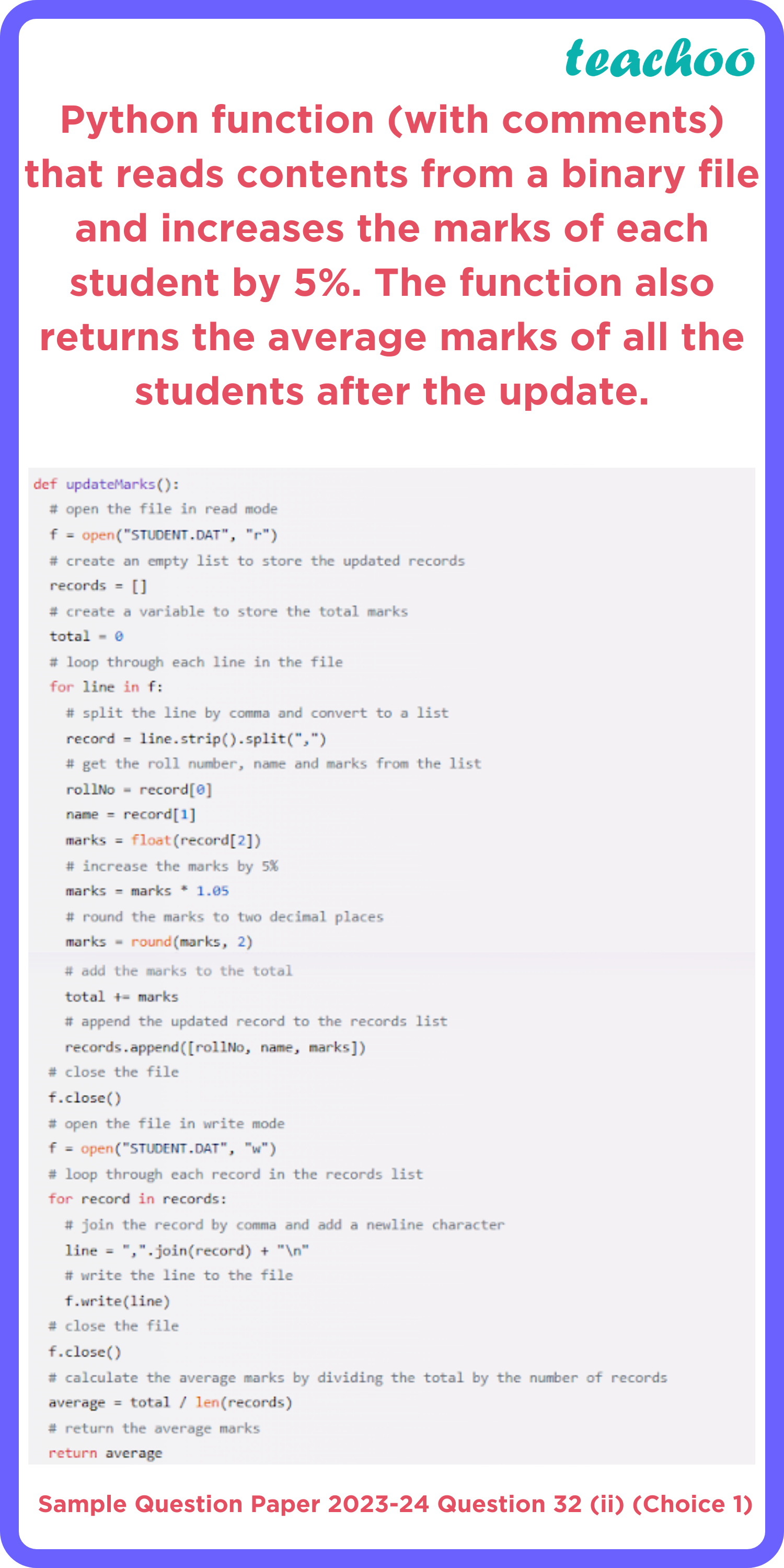Teachoo Sample Paper - Computer Science Class 12

Computer Science - Class 12
Solutions to CBSE Sample Paper - Computer Science Class 12

## Write a function, updateMarks(), that reads contents from the file STUDENT.DAT and increases the marks of each student by 5%. The function should return the average marks of all the students after the update.• The question asks us to write a function, updateMarks(), that reads contents from a file, STUDENT.DAT, and increases the marks of each student by 5%. The function should also return the average marks of all the students after the update.
• To solve this problem, we need to perform the following steps:
• Open the file in read mode using the open() function with “r” as the mode argument. This will allow us to read data from the file.
• Create an empty list to store the updated records. We will use this list to write back to the file later.
• Create a variable to store the total marks of all the students. We will use this variable to calculate the average marks later.
• Loop through each line in the file using a for loop. Each line represents a record of a student with their roll number, name and marks separated by commas.
• Split the line by comma and convert it to a list using the split() method. This will give us a list of three elements: roll number, name and marks.
• Get the roll number, name and marks from the list by indexing. The roll number is at index 0, name is at index 1 and marks is at index 2. We need to convert the marks from string to float using the float() function, as we need to perform arithmetic operations on it.
• Increase the marks by 5% by multiplying it with 1.05. This is equivalent to adding 5% of the original marks to it.
• Round the marks to two decimal places using the round() function. This will make sure that we do not have more than two digits after the decimal point.
• Add the marks to the total variable using += operator. This will keep track of the sum of all the updated marks.
• Append the updated record to the records list using append() method. The updated record should be a list of three elements: roll number, name and marks.
• Close the file using close() method. This will free up any resources used by the file object.
• Open the file again in write mode using open() function with “w” as the mode argument. This will allow us to write data to the file. Note that this will overwrite any existing data in the file, so we need to make sure that we have stored all the updated records in our records list before doing this step.
• Loop through each record in our records list using another for loop.
• Join each record by comma and add a newline character at the end using join() method. This will give us a string that represents a line in our file format.
• Write this string to our file using write() method. This will add this line to our file.
• Close our file again using close() method.
• Calculate the average marks by dividing the total marks by the number of records using / operator. We can get the number of records by using len() function on our records list.
• Return the average marks using return statement. This will end our function and give back the result to the caller.

The final code is:Learn in your speed, with individual attention - Teachoo Maths 1-on-1 Class